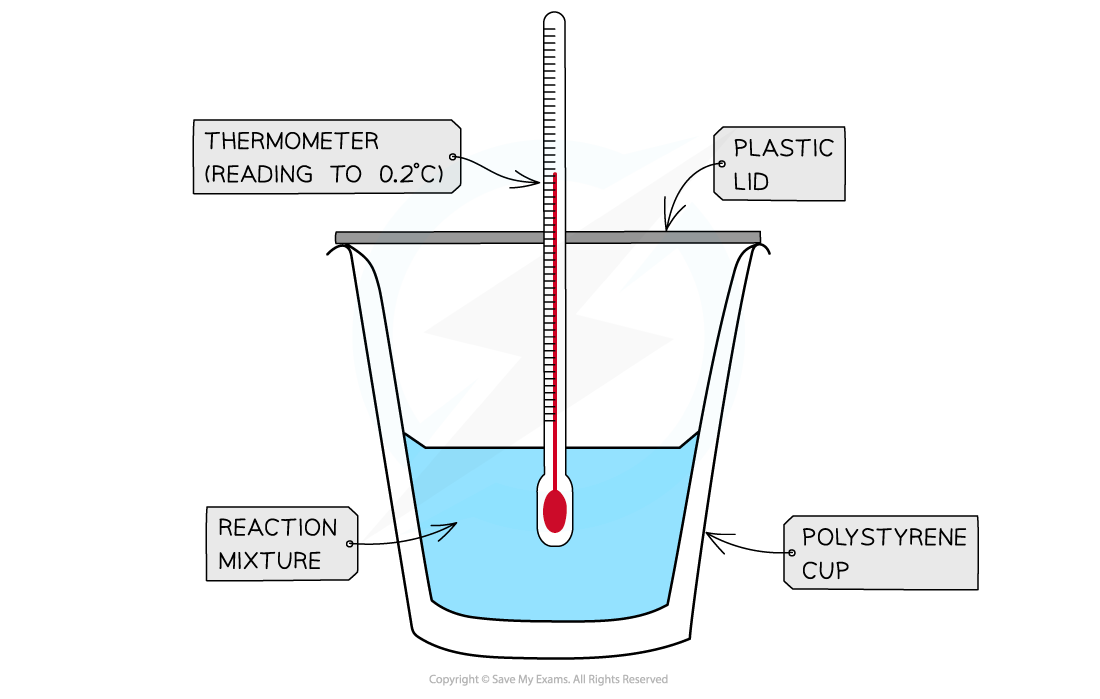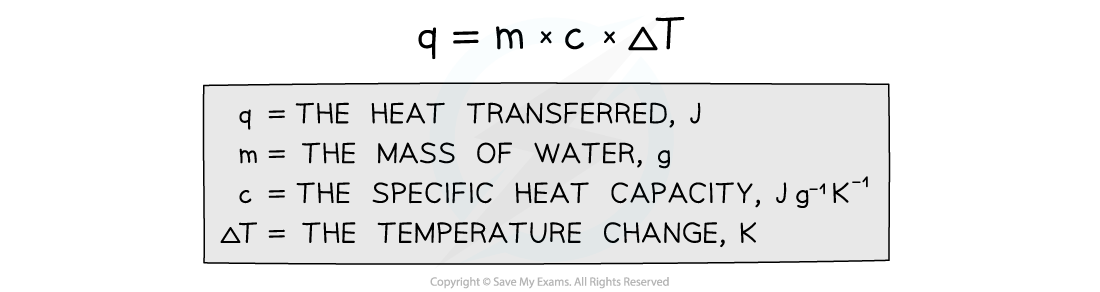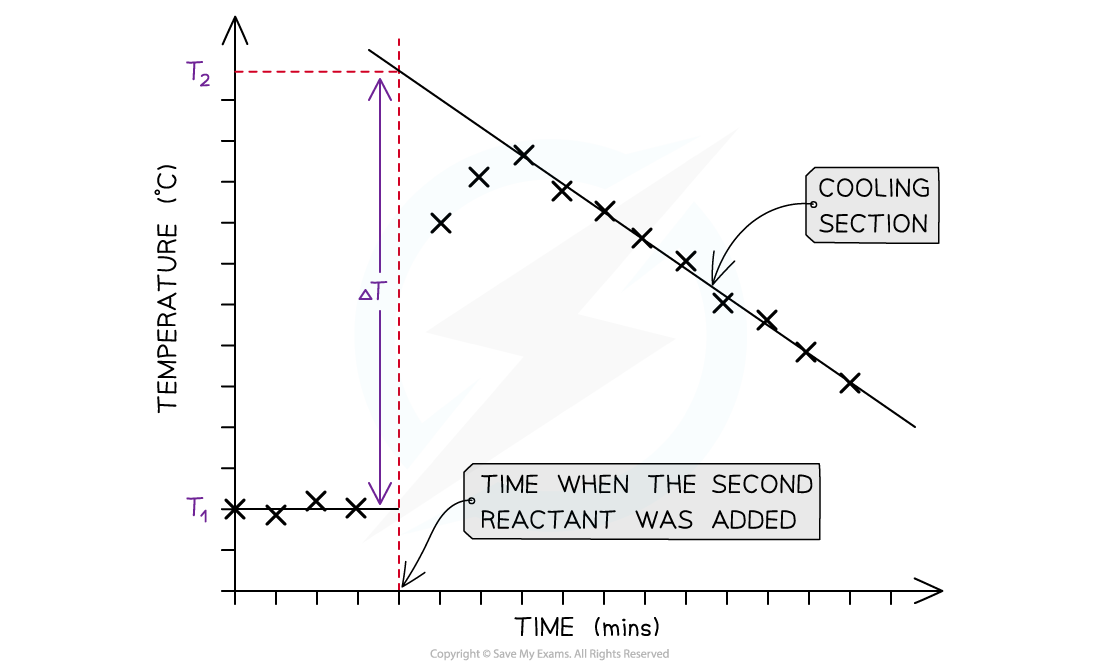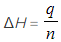# Edexcel A Level Chemistry:复习笔记4.1.4 Determining Enthalpy Change of Reaction

### Core Practical 8: Determining Enthalpy Change of Reaction

#### Measuring enthalpy changes

• Calorimetry is a technique used to measure changes in enthalpy of chemical reactions
• A calorimeter can be made up of a polystyrene drinking cup, a vacuum flask or metal canA polystyrene cup can act as a calorimeter to find enthalpy changes in a chemical reaction

• The energy needed to raise the temperature of 1 g of a substance by 1 K is called the specific heat capacity (c) of the liquid
• The specific heat capacity of water is 4.18 J g-1 K-1
• The energy transferred as heat can be calculated by:Equation for calculating energy transferred in a calorimeter

#### Enthalpy changes for reactions in solution

• The principle of these calorimetry experiments is to carry out the reaction with an excess of one reagent and measure the temperature change over the course of a few minutes
• The apparatus needed to carry out an enthalpy of reaction in solution calorimetry experiment is shown above

Sample method for a displacement reaction

1. Using a measuring cylinder place 25 cm3 of the 1.0 mol dm-3 copper(II) sulphate solution into the polystyrene cup
2. Weigh about 6 g of zinc powder - as this is an excess there is no need to be very accurate
3. Draw a table to record the initial temperature and then the temperature and time every half minute up to 9.5 minutes
4. Put a thermometer or temperature probe in the cup, stir, and record the temperature every half minute for 2.5 minutes
5. At precisely 3 minutes, add the zinc powder to the cup (DO NOT RECORD THE TEMPERATURE AT 3 MINUTES)
6. Continue stirring and record the temperature for an additional 6 minutes
• For the purposes of the calculations, some assumptions are made about the experiment:
• That the specific heat capacity of the solution is the same as pure water, i.e. 4.18 J g-1 K-1
• That the density of the solution is the same as pure water, i.e. 1 g cm-3
• The specific heat capacity of the container is ignored
• The reaction is complete
• There are negligible heat losses

#### Temperature correction graphs

• For reactions which are not instantaneous there may be a delay before the maximum temperature is reached
• During that delay the substances themselves may be losing heat to the surroundings, so that the true maximum temperature is never actually reached
• To overcome this problem we can use graphical analysis to determine the maximum enthalpy changeA temperature correction graph for a metal displacement reaction between zinc and copper sulfate solution. The zinc is added after 4 minutes

The steps to make a temperature correction graph are:

1. Take a temperature reading before adding the reactants for a few minutes to get a steady value
2. Add the second reactant and continue recording the temperature and time
3. Plot the graph and extrapolate the cooling part of the graph until you intersect the time at which the second reactant was added

#### Analysis

• Use both extrapolated lines to calculate ΔT as shown on the graph
• Use the equation q  = mcΔT to calculate the energy transferred
• q = energy transferred
• m = mass - this will be the mass of the 25 cm3 solution which will be 25 g (assuming a density of 1 g cm-3)
• c = specific heat capacity - this will be assumed to be 4.18 J g-1 K-1, which is the specific heat capacity of water
• ΔT = the temperature change from the graph
• Convert your value for energy transferred from J into kJ
• Then use the equationto calculate the enthalpy change for the reaction
• q = energy transferred
• n = number of moles - this would be the number of moles of the limiting reagent, which means that you will have an extra calculation to do to determine whether this is the zinc or the copper sulfate
• Remember that in the example above, the temperature of the reaction mixture increased which means that the reaction is exothermic and should, therefore, have a negative value